Скачать презентацию Applied Cryptography Public Key RSA Public Key

• Количество слайдов: 33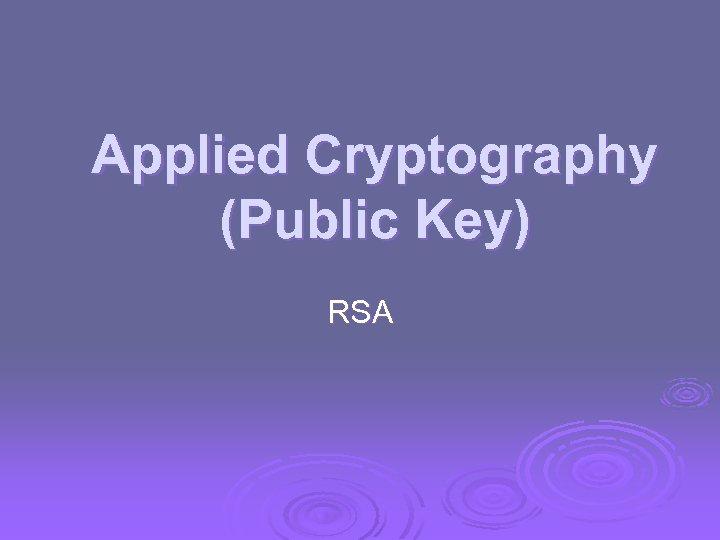Applied Cryptography (Public Key) RSA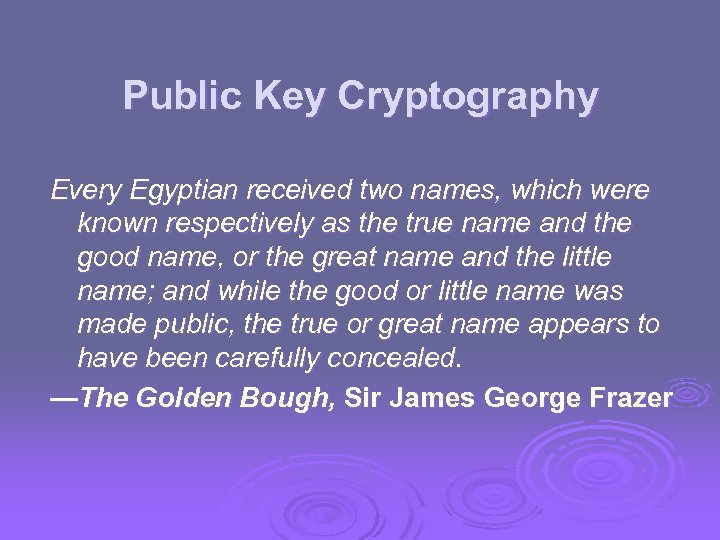Public Key Cryptography Every Egyptian received two names, which were known respectively as the true name and the good name, or the great name and the little name; and while the good or little name was made public, the true or great name appears to have been carefully concealed. —The Golden Bough, Sir James George Frazer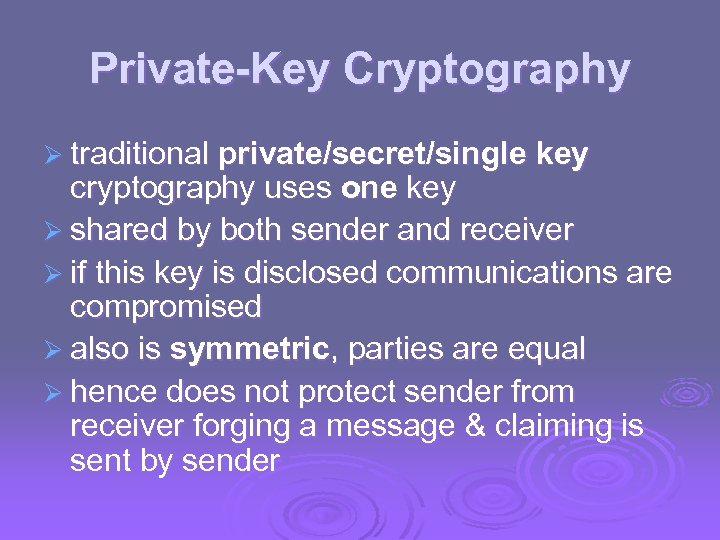Private-Key Cryptography Ø traditional private/secret/single key cryptography uses one key Ø shared by both sender and receiver Ø if this key is disclosed communications are compromised Ø also is symmetric, parties are equal Ø hence does not protect sender from receiver forging a message & claiming is sent by sender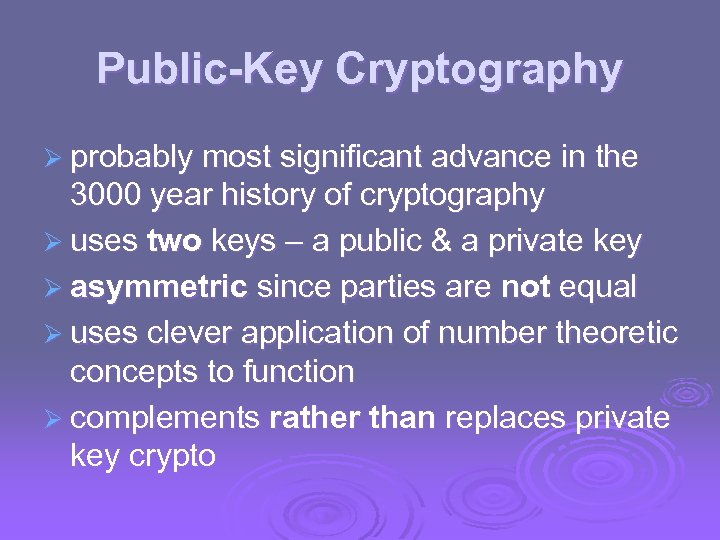Public-Key Cryptography Ø probably most significant advance in the 3000 year history of cryptography Ø uses two keys – a public & a private key Ø asymmetric since parties are not equal Ø uses clever application of number theoretic concepts to function Ø complements rather than replaces private key crypto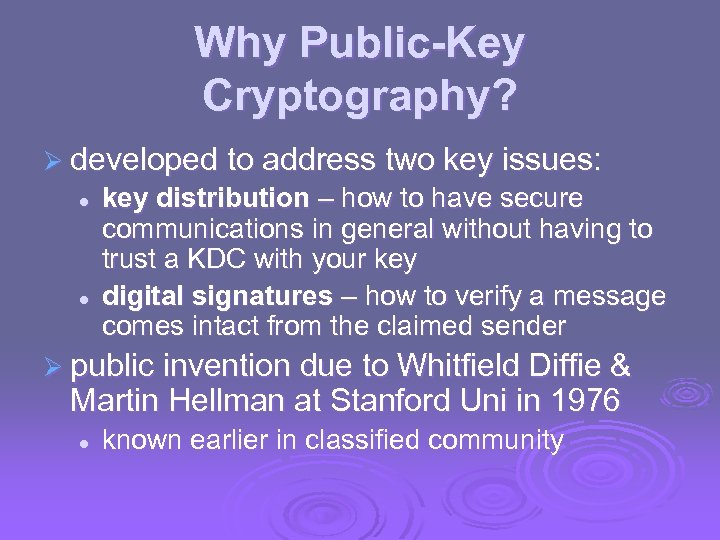Why Public-Key Cryptography? Ø developed to address two key issues: l l key distribution – how to have secure communications in general without having to trust a KDC with your key digital signatures – how to verify a message comes intact from the claimed sender Ø public invention due to Whitfield Diffie & Martin Hellman at Stanford Uni in 1976 l known earlier in classified community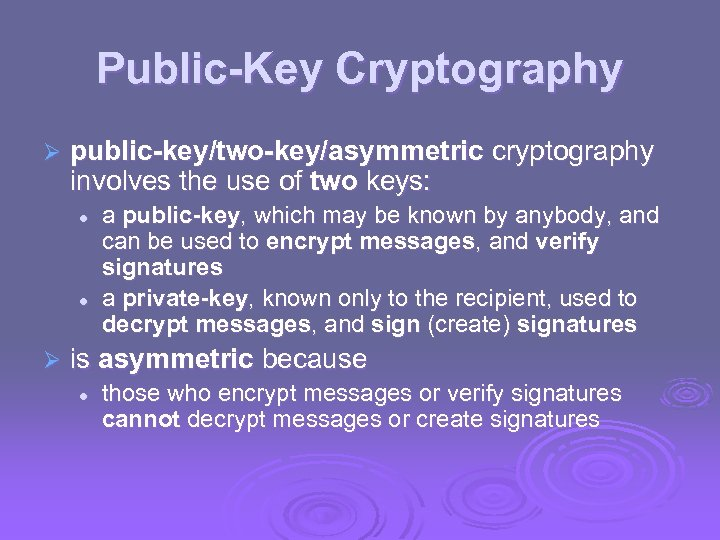Public-Key Cryptography Ø public-key/two-key/asymmetric cryptography involves the use of two keys: l l Ø a public-key, which may be known by anybody, and can be used to encrypt messages, and verify signatures a private-key, known only to the recipient, used to decrypt messages, and sign (create) signatures is asymmetric because l those who encrypt messages or verify signatures cannot decrypt messages or create signatures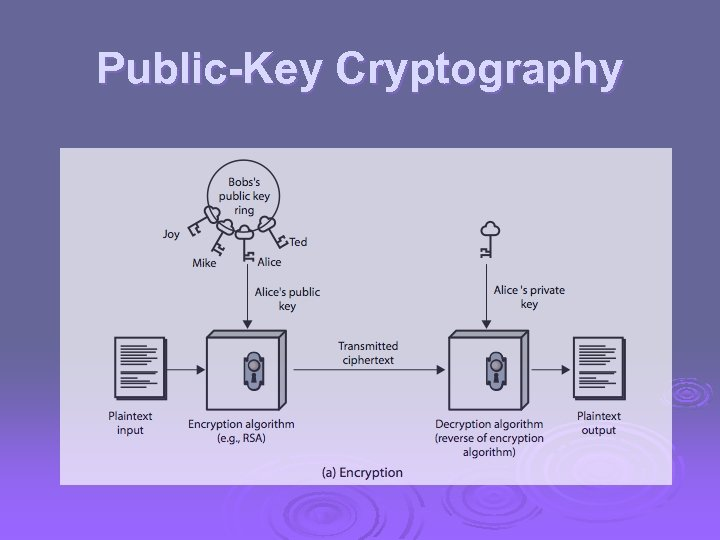Public-Key Cryptography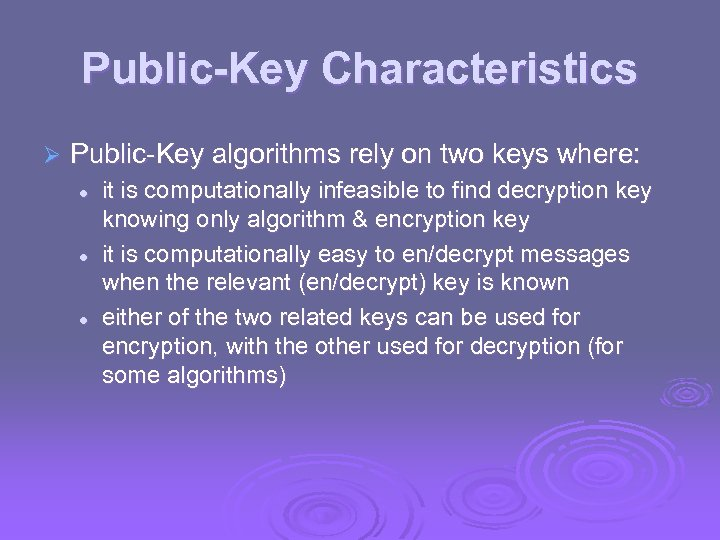Public-Key Characteristics Ø Public-Key algorithms rely on two keys where: l l l it is computationally infeasible to find decryption key knowing only algorithm & encryption key it is computationally easy to en/decrypt messages when the relevant (en/decrypt) key is known either of the two related keys can be used for encryption, with the other used for decryption (for some algorithms)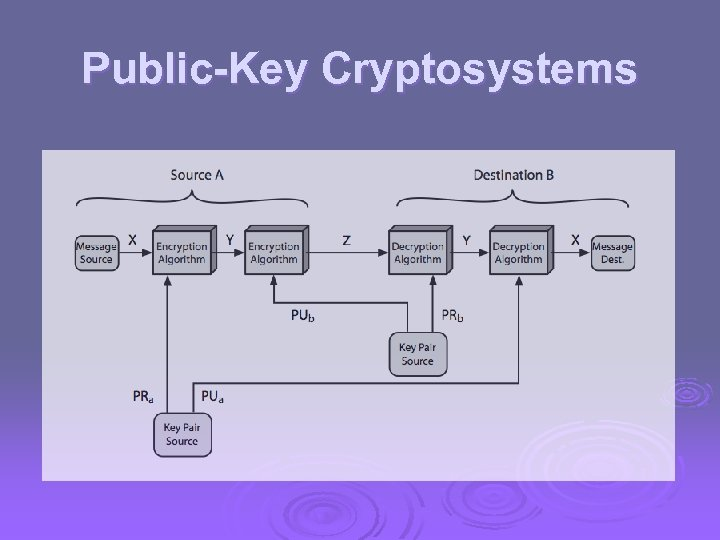Public-Key Cryptosystems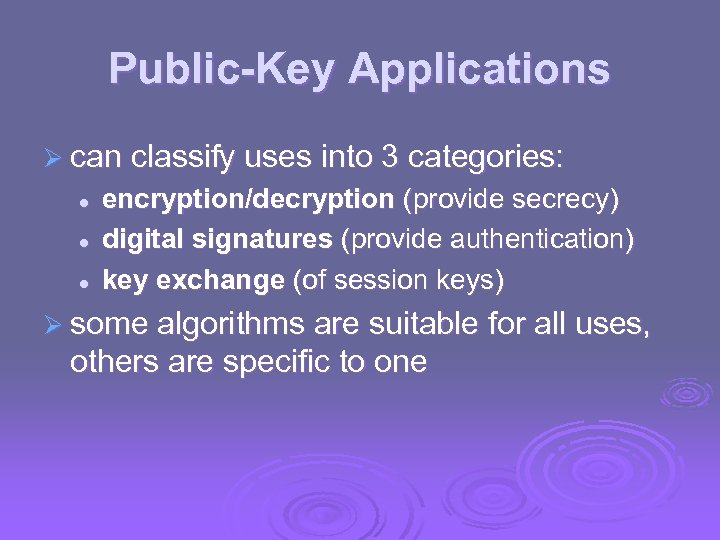Public-Key Applications Ø can classify uses into 3 categories: l l l encryption/decryption (provide secrecy) digital signatures (provide authentication) key exchange (of session keys) Ø some algorithms are suitable for all uses, others are specific to one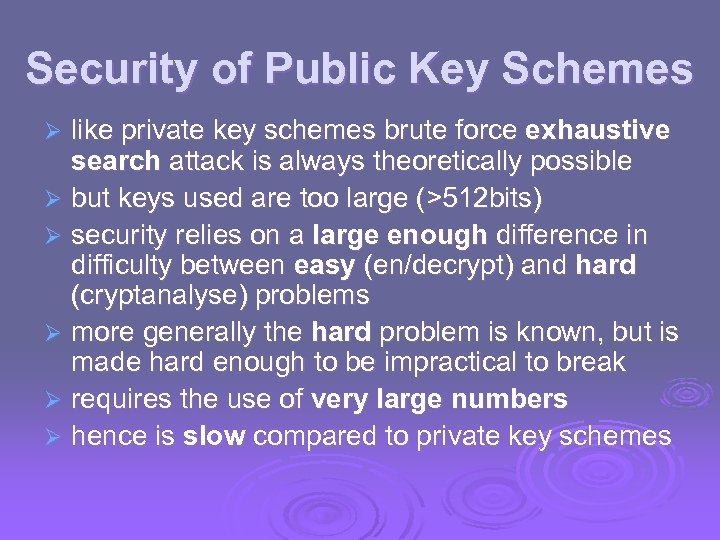Security of Public Key Schemes like private key schemes brute force exhaustive search attack is always theoretically possible Ø but keys used are too large (>512 bits) Ø security relies on a large enough difference in difficulty between easy (en/decrypt) and hard (cryptanalyse) problems Ø more generally the hard problem is known, but is made hard enough to be impractical to break Ø requires the use of very large numbers Ø hence is slow compared to private key schemes Ø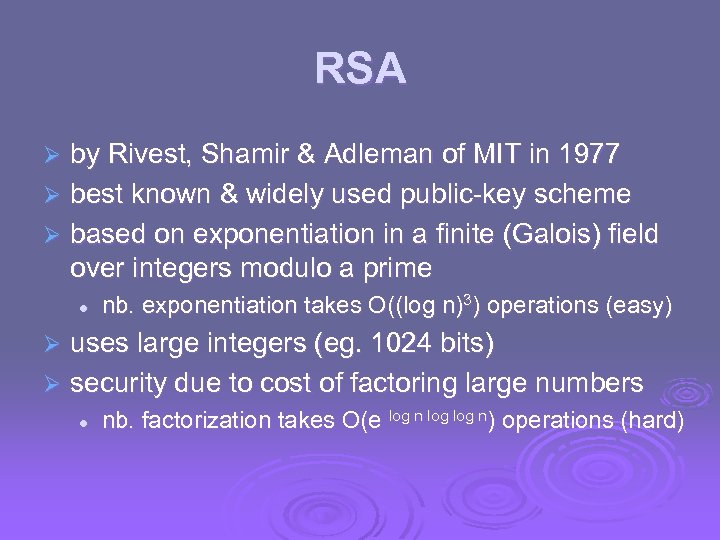RSA by Rivest, Shamir & Adleman of MIT in 1977 Ø best known & widely used public-key scheme Ø based on exponentiation in a finite (Galois) field over integers modulo a prime Ø l nb. exponentiation takes O((log n)3) operations (easy) uses large integers (eg. 1024 bits) Ø security due to cost of factoring large numbers Ø l nb. factorization takes O(e log n) operations (hard)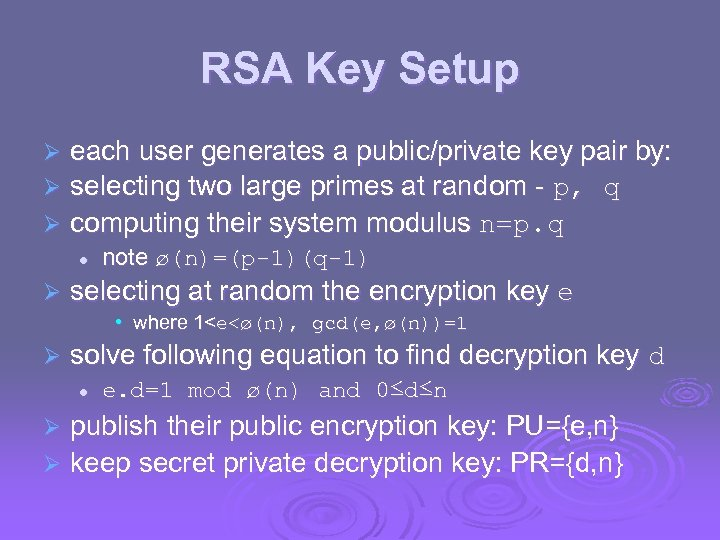RSA Key Setup each user generates a public/private key pair by: selecting two large primes at random - p, q Ø computing their system modulus n=p. q Ø Ø l Ø note ø(n)=(p-1)(q-1) selecting at random the encryption key e • where 1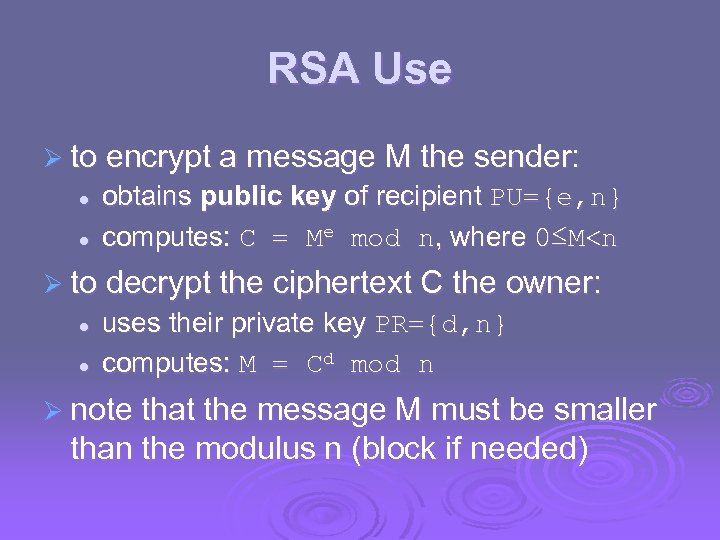RSA Use Ø to encrypt a message M the sender: l l obtains public key of recipient PU={e, n} computes: C = Me mod n, where 0≤M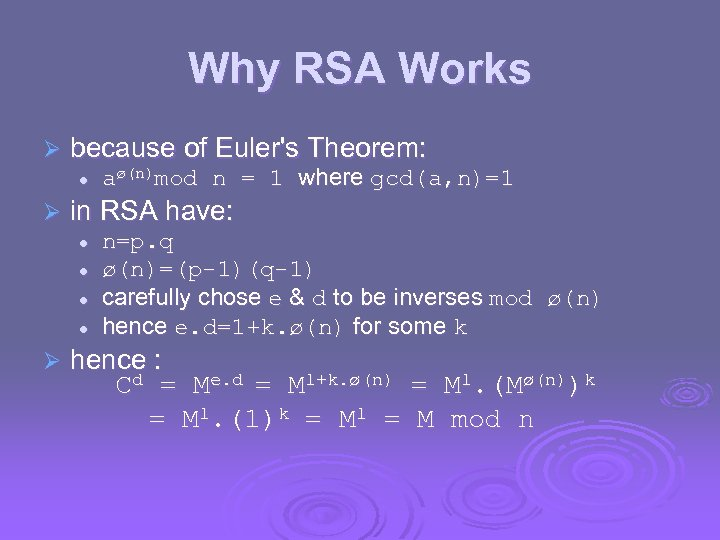Why RSA Works Ø because of Euler's Theorem: l Ø in RSA have: l l Ø aø(n)mod n = 1 where gcd(a, n)=1 n=p. q ø(n)=(p-1)(q-1) carefully chose e & d to be inverses mod ø(n) hence e. d=1+k. ø(n) for some k hence : Cd = Me. d = M 1+k. ø(n) = M 1. (Mø(n))k = M 1. (1)k = M 1 = M mod n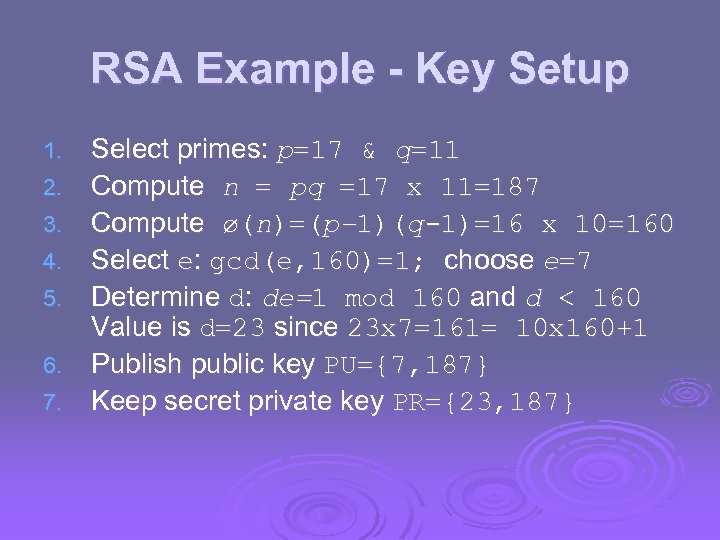RSA Example - Key Setup 1. 2. 3. 4. 5. 6. 7. Select primes: p=17 & q=11 Compute n = pq =17 x 11=187 Compute ø(n)=(p– 1)(q-1)=16 x 10=160 Select e: gcd(e, 160)=1; choose e=7 Determine d: de=1 mod 160 and d < 160 Value is d=23 since 23 x 7=161= 10 x 160+1 Publish public key PU={7, 187} Keep secret private key PR={23, 187}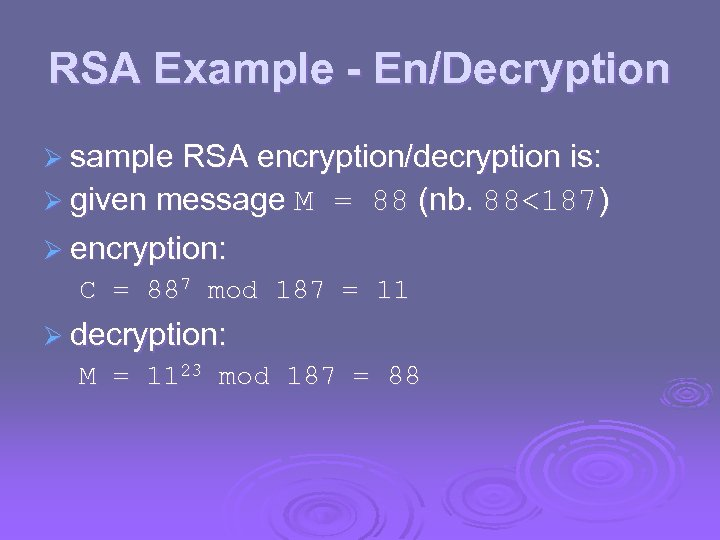RSA Example - En/Decryption Ø sample RSA encryption/decryption is: Ø given message M = 88 (nb. 88<187) Ø encryption: C = 887 mod 187 = 11 Ø decryption: M = 1123 mod 187 = 88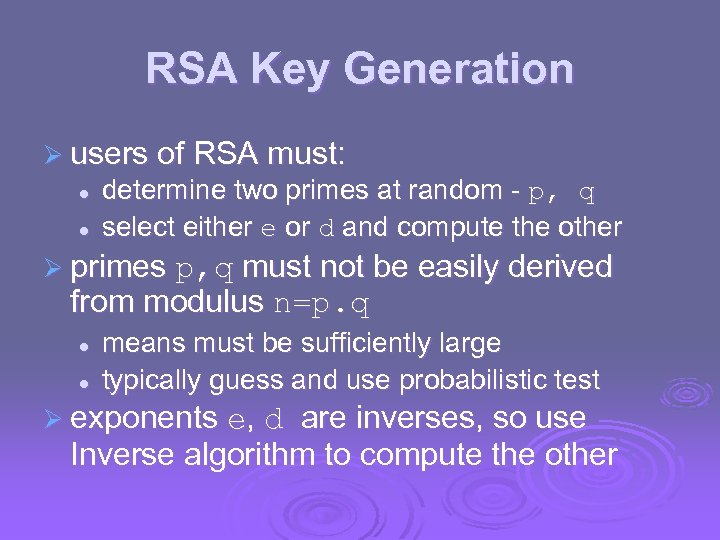RSA Key Generation Ø users of RSA must: l l determine two primes at random - p, q select either e or d and compute the other Ø primes p, q must not be easily derived from modulus n=p. q l l means must be sufficiently large typically guess and use probabilistic test Ø exponents e, d are inverses, so use Inverse algorithm to compute the other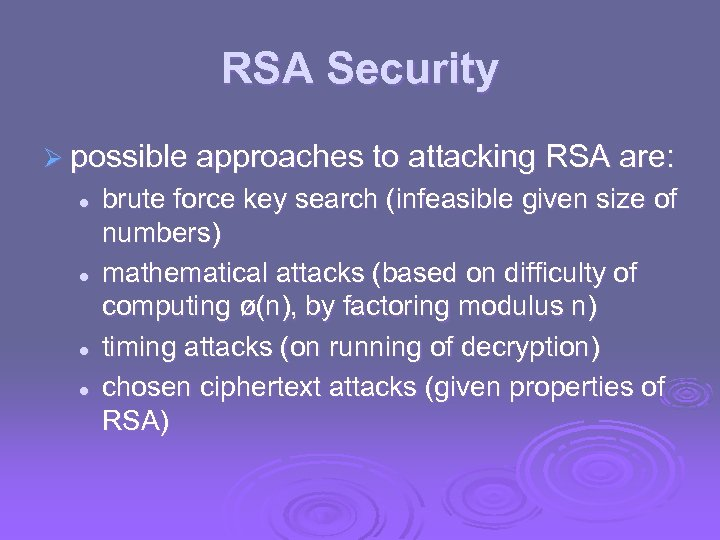RSA Security Ø possible approaches to attacking RSA are: l l brute force key search (infeasible given size of numbers) mathematical attacks (based on difficulty of computing ø(n), by factoring modulus n) timing attacks (on running of decryption) chosen ciphertext attacks (given properties of RSA)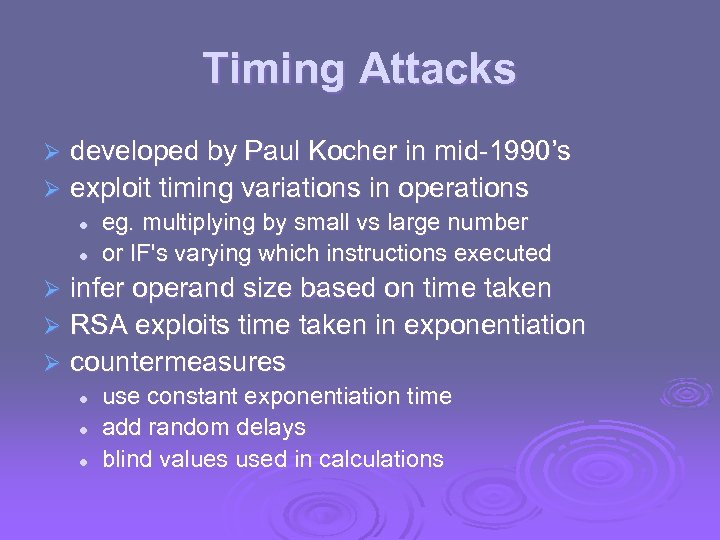Timing Attacks developed by Paul Kocher in mid-1990’s Ø exploit timing variations in operations Ø l l eg. multiplying by small vs large number or IF's varying which instructions executed infer operand size based on time taken Ø RSA exploits time taken in exponentiation Ø countermeasures Ø l l l use constant exponentiation time add random delays blind values used in calculations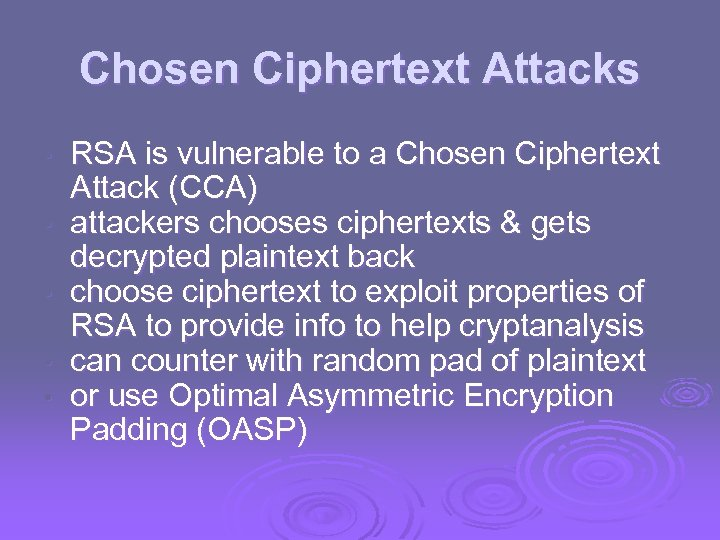Chosen Ciphertext Attacks • • • RSA is vulnerable to a Chosen Ciphertext Attack (CCA) attackers chooses ciphertexts & gets decrypted plaintext back choose ciphertext to exploit properties of RSA to provide info to help cryptanalysis can counter with random pad of plaintext or use Optimal Asymmetric Encryption Padding (OASP)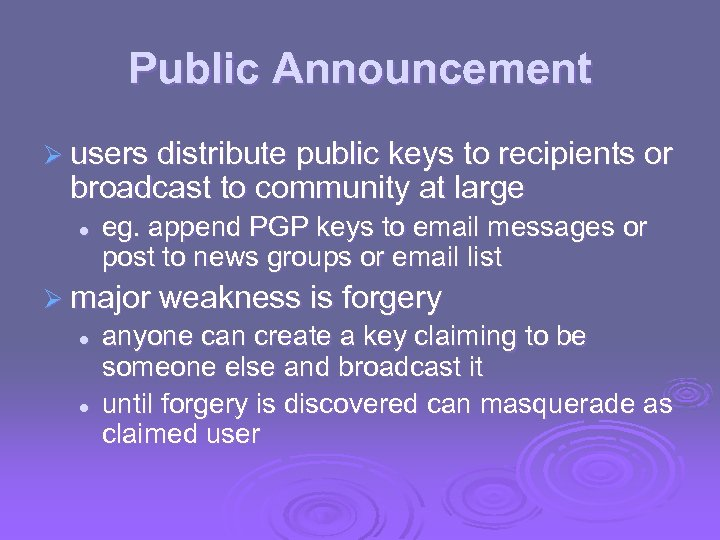Public Announcement Ø users distribute public keys to recipients or broadcast to community at large l eg. append PGP keys to email messages or post to news groups or email list Ø major weakness is forgery l l anyone can create a key claiming to be someone else and broadcast it until forgery is discovered can masquerade as claimed user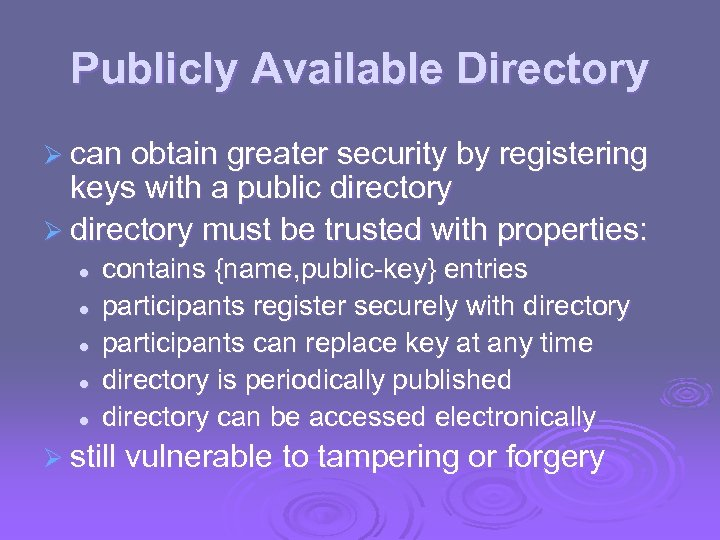Publicly Available Directory Ø can obtain greater security by registering keys with a public directory Ø directory must be trusted with properties: l l l contains {name, public-key} entries participants register securely with directory participants can replace key at any time directory is periodically published directory can be accessed electronically Ø still vulnerable to tampering or forgery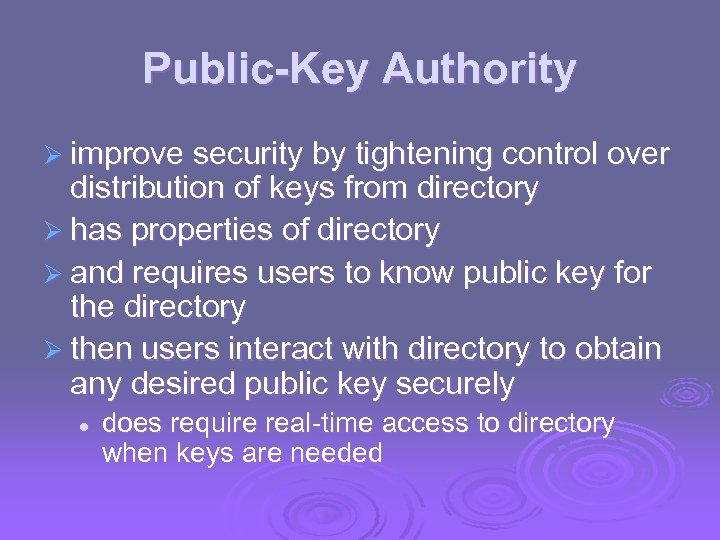Public-Key Authority Ø improve security by tightening control over distribution of keys from directory Ø has properties of directory Ø and requires users to know public key for the directory Ø then users interact with directory to obtain any desired public key securely l does require real-time access to directory when keys are needed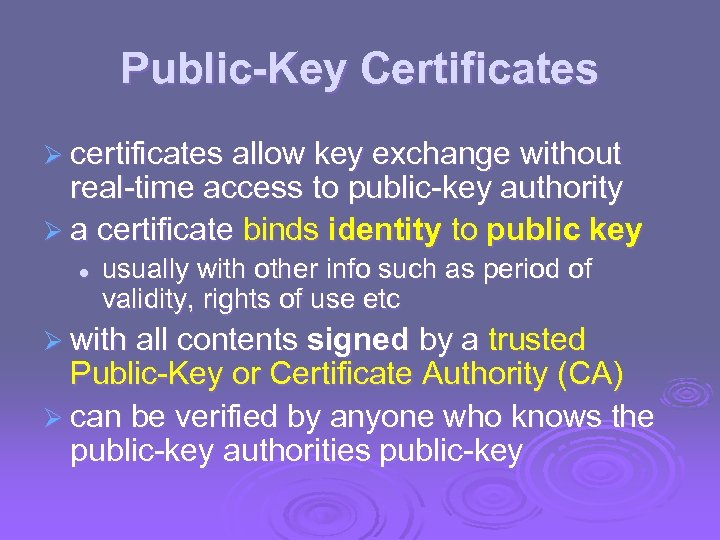Public-Key Certificates Ø certificates allow key exchange without real-time access to public-key authority Ø a certificate binds identity to public key l usually with other info such as period of validity, rights of use etc Ø with all contents signed by a trusted Public-Key or Certificate Authority (CA) Ø can be verified by anyone who knows the public-key authorities public-key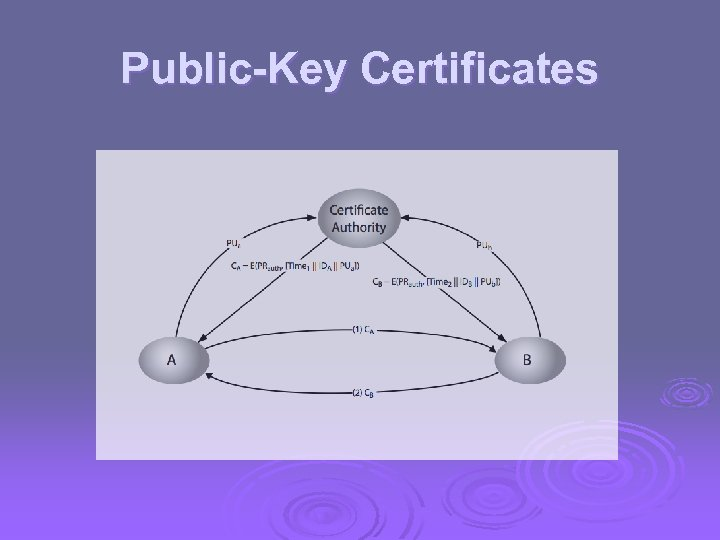Public-Key Certificates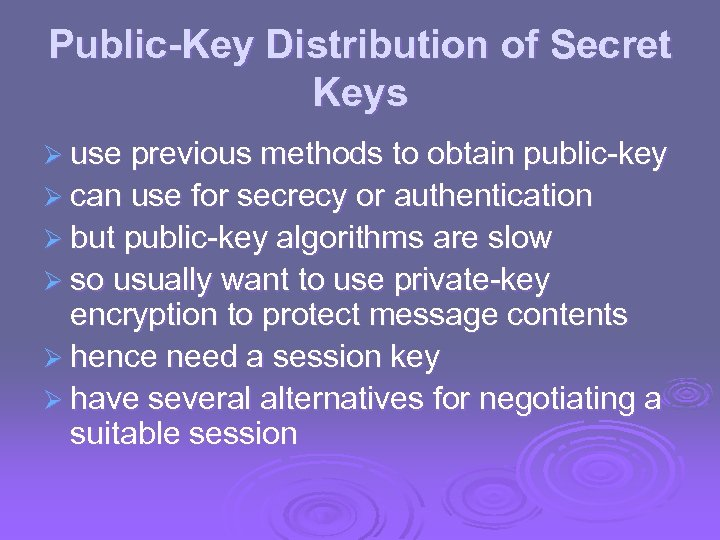Public-Key Distribution of Secret Keys Ø use previous methods to obtain public-key Ø can use for secrecy or authentication Ø but public-key algorithms are slow Ø so usually want to use private-key encryption to protect message contents Ø hence need a session key Ø have several alternatives for negotiating a suitable session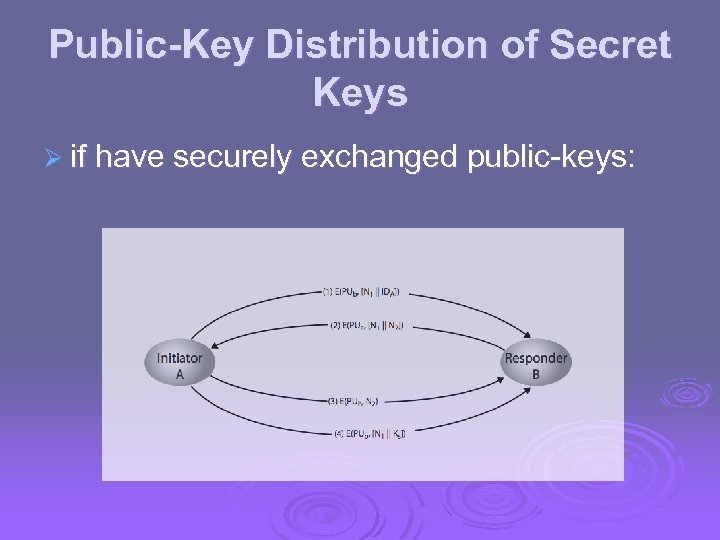Public-Key Distribution of Secret Keys Ø if have securely exchanged public-keys: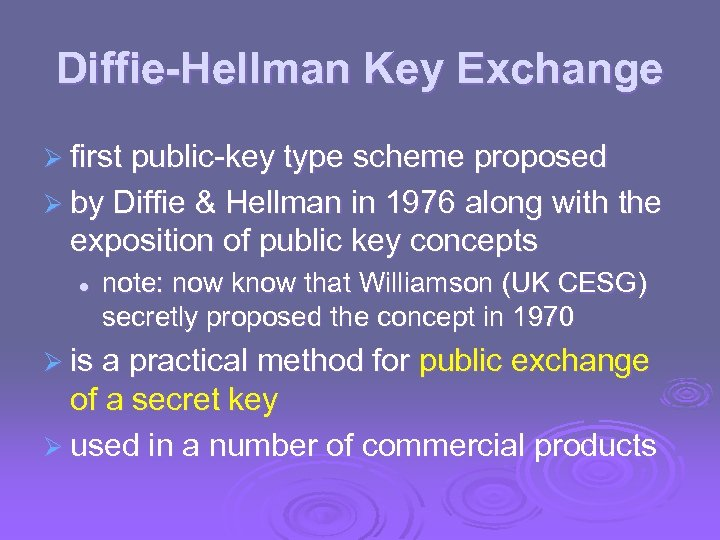Diffie-Hellman Key Exchange Ø first public-key type scheme proposed Ø by Diffie & Hellman in 1976 along with the exposition of public key concepts l note: now know that Williamson (UK CESG) secretly proposed the concept in 1970 Ø is a practical method for public exchange of a secret key Ø used in a number of commercial products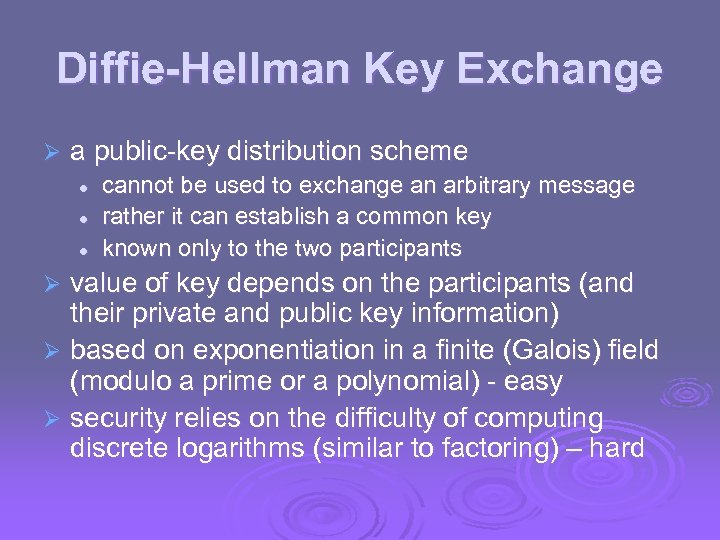Diffie-Hellman Key Exchange Ø a public-key distribution scheme l l l cannot be used to exchange an arbitrary message rather it can establish a common key known only to the two participants value of key depends on the participants (and their private and public key information) Ø based on exponentiation in a finite (Galois) field (modulo a prime or a polynomial) - easy Ø security relies on the difficulty of computing discrete logarithms (similar to factoring) – hard Ø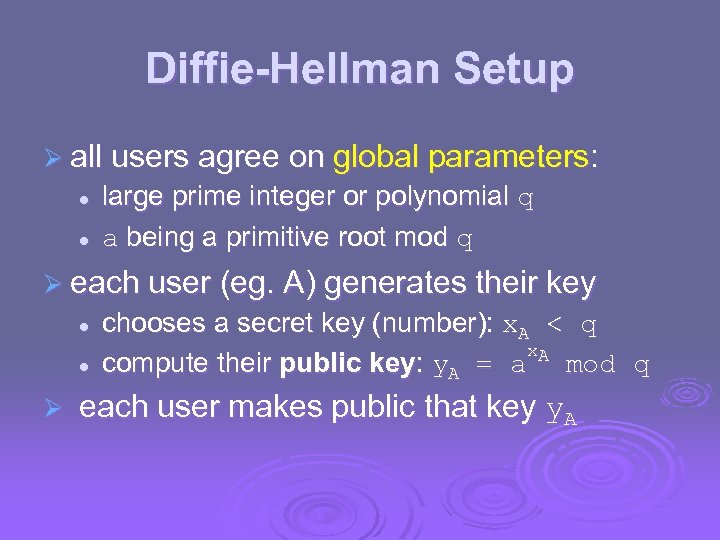Diffie-Hellman Setup Ø all users agree on global parameters: l l large prime integer or polynomial q a being a primitive root mod q Ø each user (eg. A) generates their key l l Ø chooses a secret key (number): x. A < q x. A compute their public key: y. A = a mod q each user makes public that key y. A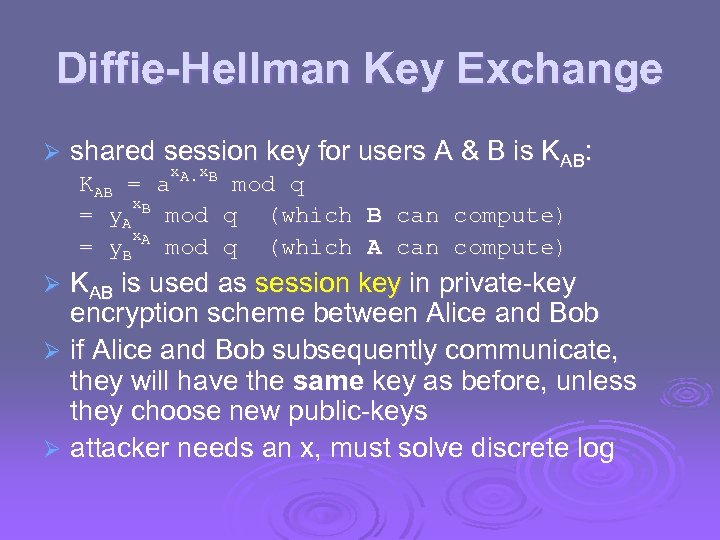Diffie-Hellman Key Exchange Ø shared session key for users A & B is KAB: x x KAB = a A. B mod q x. B = y. A mod q (which B can compute) x. A = y. B mod q (which A can compute) KAB is used as session key in private-key encryption scheme between Alice and Bob Ø if Alice and Bob subsequently communicate, they will have the same key as before, unless they choose new public-keys Ø attacker needs an x, must solve discrete log Ø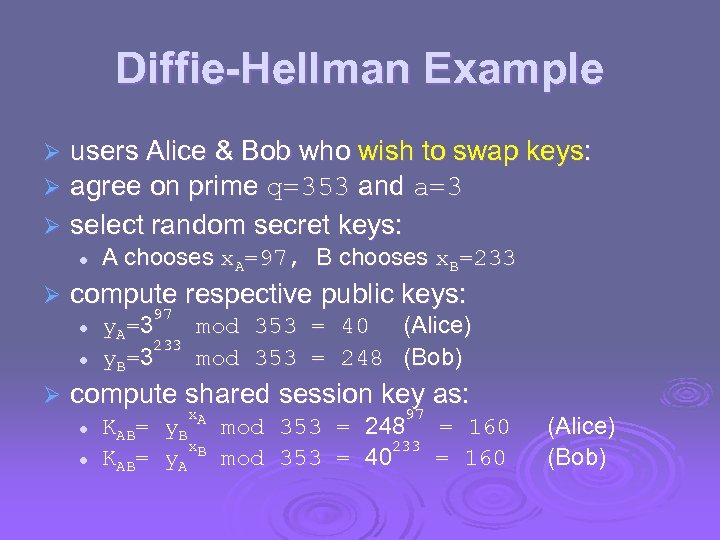Diffie-Hellman Example users Alice & Bob who wish to swap keys: agree on prime q=353 and a=3 Ø select random secret keys: Ø Ø l Ø compute respective public keys: l l Ø A chooses x. A=97, B chooses x. B=233 97 y A= 3 mod 353 = 40 (Alice) 233 y B= 3 mod 353 = 248 (Bob) compute shared session key as: l l KAB= x. A y. B x. B y. A 97 mod 353 = 248 = 160 233 mod 353 = 40 = 160 (Alice) (Bob)